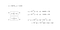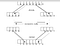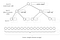# Algorithms Illuminated

Algorithms Illuminated, by Tim Roughgarden, professor at Columbia University,is a 4 part book series inspired by his online courses on Coursera and EdX. His video lectures are also available on YouTube.

Here, I will review the first book in the four-part series:

PART-1: The Basics

PART-2: Graph Algorithms and Data Structures

PART-3: Greedy Algorithms and Dynamic Programming

PART-4: Algorithms for NP-Hard Problems

Part 1- provides an introduction to:

• Algorithms
• Asymptotic Analysis
• Divide and Conquer Algorithms
• Master Method
• Sorting and Selection

This book helps you in sharpening your analytical skills and thinking algorithmically.

This book has quizzes, end of chapter problems, and programming problems to evaluate what you’ve learnt.

# Algorithms

## Why study Algorithms?

1. Understanding the basics of algorithms is essential for doing serious work in the field of computer science.
2. Algorithms play a key role in modern technological innovations.

This book follows a pattern of first introducing a computational problem and then describing one or more algorithms that solve the problem.

We begin by integer multiplication of 2 n-digit numbers, solving it using the grade school algorithm (the way we used to solve in school). We assess the performance of the algorithm through the number of primitive operations (primitive operations include simple tasks like adding, comparing or copying) it performs, as a function of the number of digits n in each input number. The primitive operations that we perform in this problem are addition and multiplication. The running time of this algorithm is O(n²). And recursive integer multiplication makes 4 recursive calls.

“Perhaps the most important principle for the good algorithm designer is to refuse to be content.”

So, we explore another algorithm called Karatsuba Multiplication which is faster than the grade school algorithm. In the Karatsuba algorithm, we divide the numbers into halves and compute as follows:Karatsuba Multiplication

Here in Karatsuba multiplication, we make only 3 recursive calls and get running time as O(n^1.59), which is better than grade school algorithm.

Merge Sort Algorithm

Sorting is the process of arranging data in a particular format. We’ve many sorting algorithms, like selection sort, insertion sort and bubble sort. All these algorithms have quadratic running times i.e. O(n²). Our quest to answer the question ‘Can we do better?’ leads us to merge sort.

This is a canonical example of divide-and-conquer algorithm , that splits the input array into 2 halves, recursively sorts each half, and combines the result.Merge Sort Algorithm

This tree structure provides us with a principled way to tally the work done by merge sort across all it’s recursive calls.A recursion tree for merge sort.

In simple words, the running time of merge sort is: a loop performs n operations and the number of operations performed in dividing the input array into two n/2 arrays till one element remains in the array is log n, so the running time is n log n after ignoring the lower order terms and constant factors.

Prior to reading this book I knew what the time complexity of merge sort was. But only after reading this book I understood why is it so?

We calculate the total operations performed by merge sort as

=work per level*no. of levels ≤ 6n log n+6n

no. of levels =log n +1 (as keep on dividing the input array into 2 n/2 arrays we get log n + level 0 (the actual input)).

work per level ≤ 6n ( a valid upper bound on the number of operations performed by merge subroutine).

Three guiding principles for the analysis of algorithms are:

1. Worst-case Analysis -where we have no assumptions of the input
2. Big-picture Analysis-where we ignore lowest order terms and constant factors.
3. Asymptotic Analysis-helps us differentiate between better and worst approaches to solve a particular problem.

# Asymptotic Notation

While analyzing the running time of an algorithm we ignore constant factors and lower order terms not because they are not important, but to make things manageable.

The following 3 asymptotic notations are mostly used :

Big-Oh Notation(O): This is used to define worst case of any algorithm -maximum time required to complete its execution.

Big-Omega Notation(Ω): This is used to define the best case of any algorithm-minimum time required for its execution.

Big-Theta Notation(θ): This is used to define average case of any algorithm-average time required for its execution.

Example: If T(n)= 4n²+3n , where T(n) is the no of operations performed by the algorithm then:

T(n)=O(n³){Worst case}

T(n)=θ(n²){Average case}

T(n)=Ω(n){Best Case}

# Divide-and-Conquer Paradigm

1. Divide the input into smaller subproblems.
2. Conquer the subproblems recursively.
3. Combine the solutions for the subproblems into a solution for the original problem.

Ex: Merge sort , Karatsuba Algorithm, Strassen’s Algorithm(divide and conquer approach for matrix multiplication), etc.

# Master Method

where a=number of recursive calls,

b=factor by which input size shrinks in recursive call,

d=exponent of work done outside recursive calls.

Master Method: If T(n) is defined by a standard recurrence, of the form

then,

Ex: Let’s calculate the running time of merge sort, the standard recurrence of merge sort is given by

`T(n) = 2T(n/2) + n`

here a=2 (there are 2 recursive calls in merge sort),

b=2 (as we divide the input array into 2 equal halves),

d=1 (as work done outside recursive calls is linear i.e. n).

a=2=b^d=2¹ (case -1) , the running time of merge sort is given by

O(n^dlogn) , here d=1

so , T(n)=O(n log n).

This is the last concept I wanted to share in this article . There were many other concepts in the book, but these were a few that caught my eye. So, I wanted to share them with you all so that it may help all those people who are not familiar with this.

I knew that these concepts are important in the field of computer science but they were a mystery to me. But after uncovering them chapter by chapter, I’m now familiar with these concepts and have understood them. I’m grateful to all the mentors who helped and encouraged me to read this book and write a review of it.

Lastly, I hope this article was helpful and worth your time.

Thank You.